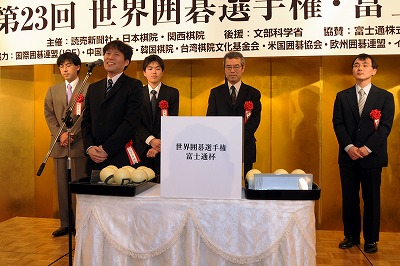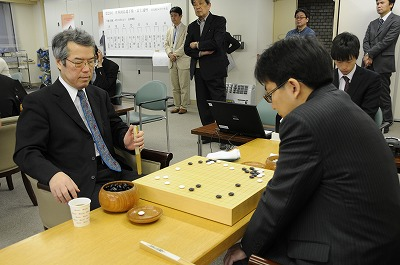## Archive for April, 2010

### Fujitsu Cup: Happiness and Desolation for Japan

Monday, April 12th, 2010

The first two rounds of the 23rd Fujitsu Cup are over.

The hosting country, Japan, started very strongly with four Japanese players winning in the first round: Anzai Nobuaki defeated Chen Shiyuan of Taiwan, Takao Shinji defeated amateur Fernando Aguilar representing South America, Hane Naoki defeated Choi Cheolhan of Korea and a very big surprise: veteran Ishida Yoshio defeated Mok Jinseok of Korea!Just like last year, though, after a strong start Japan was completely eliminated in the second round, unfortunately: Yamashita Keigo lost to Piao Wenyao of China, Cho U lost to Kim Jiseok of Korea, Anzai Nobuaki lost to Lee Sedol of Korea, Takao Shinji lost to Park Yeonghun of Korea, Hane Naoki lost to Kang Dongyun of Korea and Ishida Yoshio lost to Kong Jie of China.Here is the record of the game in which Ishida Yoshio defeated Mok Jinseok (by resignation).

(; KM[6.5] EV[23rd Fujitsu Cup 1st round] GM SZ FF PB[Ishida Yoshio] PW[Mok Jinseok] BR[9d] DT[2010-04-10] WR[9d] RE[B+R] ;B[qd];W[dc];B[dp];W[pq];B[ce];W[qn];B[oc];W[di];B[cg];W[cd];B[ed] ;W[ec];B[fd];W[be];B[dd];W[cf];B[de];W[bd];B[df];W[gb];B[bf];W[cn] ;B[co];W[dn];B[fq];W[ck];B[qi];W[jq];B[bn];W[bm];B[bo];W[qk];B[oi] ;W[op];B[kd];W[nh];B[ni];W[nd];B[nc];W[qe];B[pd];W[qg];B[ph];W[rd] ;B[rc];W[of];B[mh];W[rf];B[sd];W[mg];B[lh];W[rh];B[ri];W[re];B[mp] ;W[md];B[lb];W[qc];B[qb];W[lg];B[kg];W[kp];B[mn];W[ml];B[lm];W[ll] ;B[km];W[kl];B[jm];W[ik];B[ng];W[nf];B[pk];W[pl];B[ol];W[ok];B[nk] ;W[pj];B[nl];W[og];B[lj];W[hm];B[gk];W[hk];B[gm];W[gj];B[gl];W[hi] ;B[hl];W[mq];B[lq];W[lp];B[nq];W[mr];B[np];W[nr];B[oo];W[pp];B[ir] ;W[gp];B[fp];W[fk];B[el];W[ek];B[lr];W[or];B[ks];W[fo];B[eo];W[gn] ;B[en];W[il];B[im];W[iq];B[hr];W[fm];B[fl];W[em];B[dl];W[hn];B[dm] ;W[fn];B[cm];W[hq];B[gq];W[ho];B[fi];W[fj];B[ei];W[dk];B[do];W[dh] ;B[bi];W[fg];B[gh];W[kh];B[mm];W[lo];B[gg];W[jh];B[jl];W[kf];B[jj] ;W[ig];B[qh];W[jr];B[js];W[fh];B[gi];W[gr];B[fr];W[dg];B[gf];W[oh] ;B[pi];W[bj];B[ci];W[cj];B[ii];W[hh];B[jk];W[hj];B[hg];W[ih];B[hc] ;W[gc];B[hd];W[hb];B[jc];W[rj];B[pm];W[qm];B[jo];W[io];B[ib];W[gd] ;B[ge];W[ie];B[fc];W[fb];B[he];W[si];B[po];W[qo];B[pn];W[bl];B[ae] ;W[bb];B[aj];W[ak];B[ai];W[kr];B[gs];W[ms];B[ls];W[ha];B[oj];W[pk] ;B[ke];W[je];B[lf];W[jg];B[le];W[li];B[mi];W[ki];B[kj];W[ad];B[af] ;W[mo];B[no];W[am];B[mc];W[an];B[ao];W[id];B[ic])

Complete tournament table here.

Next round will be played in two days, and it will match four Chinese players (Gu Li, Piao Wenyao, Kong Jie and Qiu Jun) against four Korean players (Lee Sedol, Park Yeonghun, Kim Jiseok and Kang Dongyun).